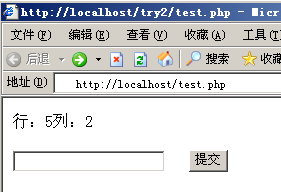# 矩阵验证的后台```<?php
include_once("c.php");
//随机生成一个行列号
\$test = rnd();
\$num1 = \$test;
\$num2 = \$test;
?>
<p>行：<?=\$num1?>列：<?=\$num2?></p>
<form id="form1" name="form1" method="POST" action="c.php">
<label>
<input type="text" name="num" id="textfield" />
<input name="rnd1" type="hidden" value="<?=\$num1?>" />
<input name="rnd2" type="hidden" value="<?=\$num2?>" />
</label>
<label>
<input type="submit" value="提交" />
</label>
</form>
```

```<?php
/*
生成一个 5 行 5 列的矩阵，为了不头脑糊涂，故让数组从1下标开始。
*/
\$pass = Array();
\$pass = 0;
\$pass = Array(0,1,2,3,4,5);
\$pass = Array(0,6,7,8,9,10);
\$pass = Array(0,11,12,13,14,15);
\$pass = Array(0,16,17,18,19,20);
\$pass = Array(0,21,22,23,24,25);
/*
函数说明：随机行列号
*/
function rnd()
{
// 产生1 到 5之间的随机数
\$rand1 = rand(1,5);
\$rand2 = rand(1,5);

// 返回一个数组，第一个元素包含行号，第二个元素包含列号
return Array(\$rand1,\$rand2);
}

// 接收行号
\$rnd1 = \$_POST['rnd1'];
// 接受列号
\$rnd2 = \$_POST['rnd2'];
// 接受对应的值
\$num = \$_POST['num'];

\$check = \$pass[\$rnd1][\$rnd2];

if ( \$num >0 )
{
if ( \$num == \$check )
echo '正确，你输入的是：'.\$num;
else
echo ':-(';
}
?>
```